# Noetherian ring

View a complete list of semi-basic definitions on this wiki
This article defines a property of commutative unital rings; a property that can be evaluated for a commutative unital ring
View all properties of commutative unital rings
VIEW RELATED: Commutative unital ring property implications | Commutative unital ring property non-implications |Commutative unital ring metaproperty satisfactions | Commutative unital ring metaproperty dissatisfactions | Commutative unital ring property satisfactions | Commutative unital ring property dissatisfactions

## Definition

Below are some equivalent definitions of Noetherian ring:

No. Shorthand A commutative unital ring is termed Noetherian if ... A commutative unital ring$R$ is termed Noetherian if ...
1 ascending chain condition on ideals any ascending chain of ideals stabilizes after a finite length arbitrary version: if$J$ is a well-ordered set and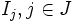$I_j, j \in J$ are ideals such that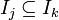$I_j \subseteq I_k$ for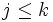$j \le k$, there exists some$j \in J$ such that$I_j = I_k$ for all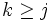$k \ge j$.
countable chain version: If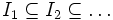$I_1 \subseteq I_2 \subseteq \dots$ is an ascending chain of ideals, then there exists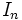$I_n$ such that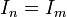$I_n = I_m$ for all$m \ge n$.
2 finite generation of ideals every ideal in the ring is finitely generated. for every ideal$I$ in$R$, there exist elements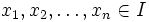$x_1, x_2, \dots, x_n \in I$ such that$I$ is the ideal generated by$x_1, x_2, \dots, x_n$.
3 finite generation of prime ideals every prime ideal in the ring is finitely generated. for every prime ideal$P$ in$R$, there exist elements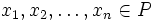$x_1, x_2, \dots, x_n \in P$ such that$P$ equals the ideal generated by$x_1, x_2, \dots, x_n$.

### Equivalence of definitions

Further information: equivalence of definitions of Noetherian ring

## Metaproperties

Metaproperty name Satisfied? Proof Statement with symbols
polynomial-closed property of commutative unital rings Yes Noetherianness is polynomial-closed Suppose$R$ is a Noetherian ring. Then, the polynomial ring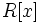$R[x]$ is also a Noetherian ring.
quotient-closed property of commutative unital rings Yes Noetherianness is quotient-closed Suppose$R$ is a Noetherian ring and$I$ is an ideal in$R$. Then, the quotient ring$R/I$ is also a Noetherian ring.
subring-closed property of commutative unital rings No Noetherianness is not subring-closed It is possible to have the following:$R$ is a Noetherian ring and$S$ is a (unital) subring of$R$, but$S$ is not a Noetherian ring. For instance, consider any non-Noetherian integral domain; this is a subring of a field, which is Noetherian.
localization-closed property of commutative unital rings Yes Noetherianness is localization-closed Suppose$R$ is a Noetherian ring and$S$ is a multiplicatively closed subset of$R$. Then, the localization of$R$ at$S$ is also Noetherian.
finite direct product-closed property of commutative unital rings Yes Noetherianness is finite direct product-closed Suppose$R_1, R_2,\dots,R_n$ are Noetherian rings. Then, the direct product$R_1 \times R_2 \times \dots \times R_n$ is also a Noetherian ring.
completion-closed property of commutative unital rings Yes Noetherianness is completion-closed Suppose$R$ is a commutative unital ring and$M$ is a maximal ideal in$R$. The completion of$R$ at$M$ is also Noetherian.

## Relation with other properties

### Conjunction with other properties

Noetherian domain integral domain
reduced Noetherian ring reduced ring: it has no nonzero nilpotent elements.
Noetherian normal domain normal domain
Noetherian unique factorization domain unique factorization domain
local Noetherian ring local ring
local Noetherian domain local domain
zero-dimensional Noetherian ring zero-dimensional ring: every prime ideal in it is a maximal ideal
one-dimensional Noetherian domain one-dimensional domain: integral domain in which every nonzero prime ideal is maximal
finite-dimensional Noetherian ring finite-dimensional ring: its Krull dimension is finite.

### Stronger properties

Property Meaning Proof of implication Proof of strictness (reverse implication failure) Intermediate notions
Polynomial ring over a field$k[x]$ for a field$k$ click here
Artinian ring descending chain of ideals stabilizes eventually Artinian implies Noetherian Noetherian not implies Artinian click here
Principal ideal ring every ideal is principal principal ideal ring implies Noetherian Noetherian not implies principal ideal ring click here